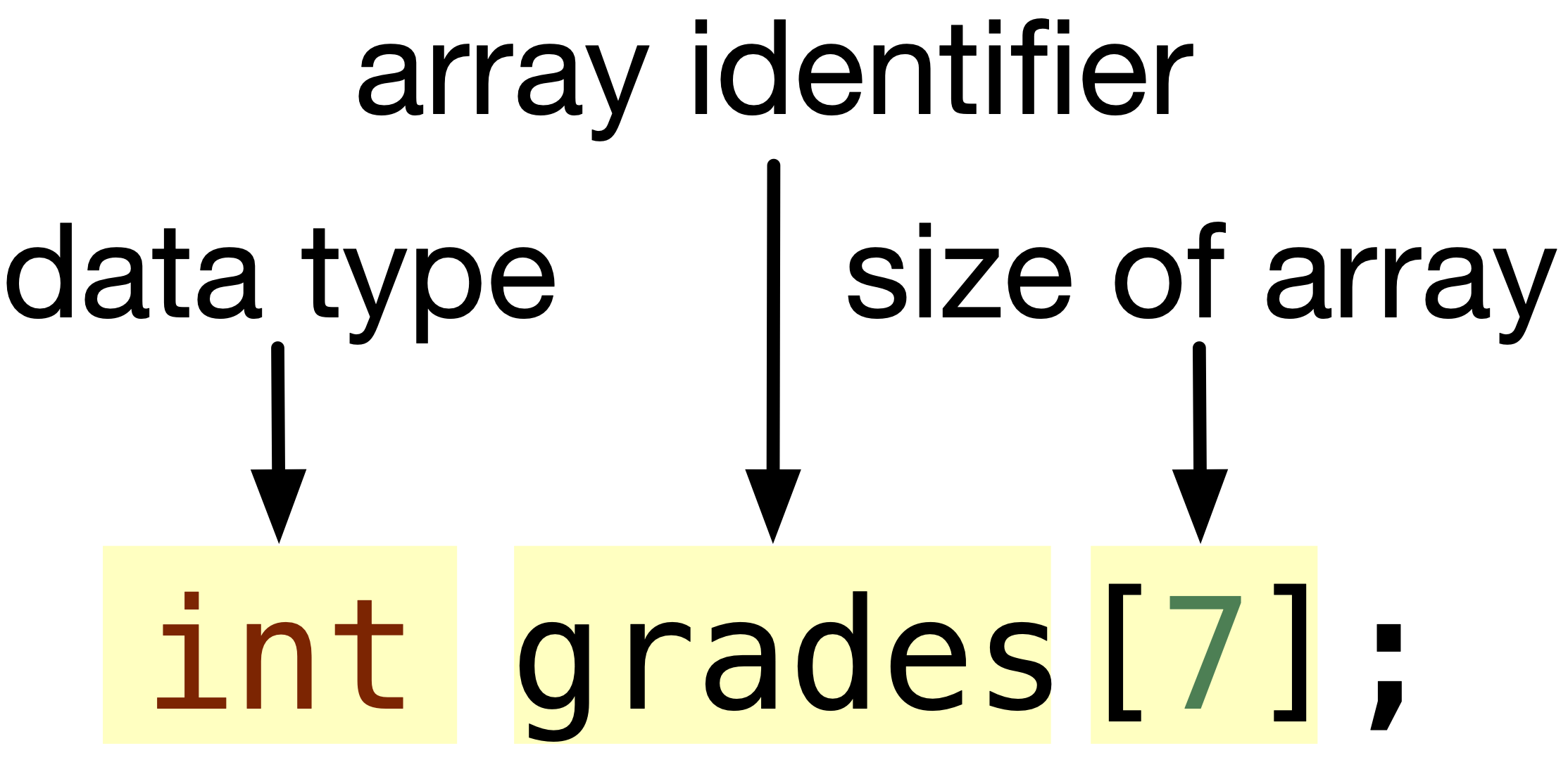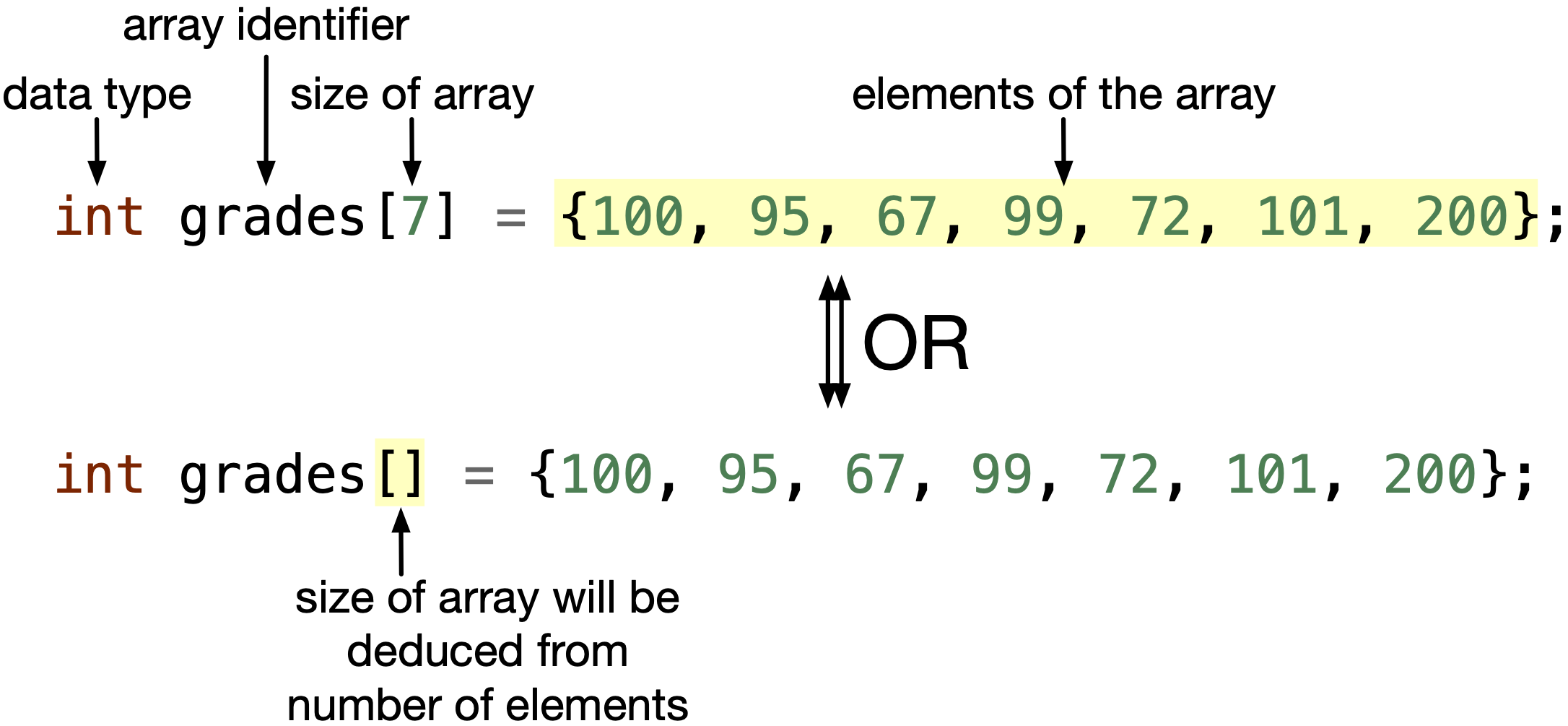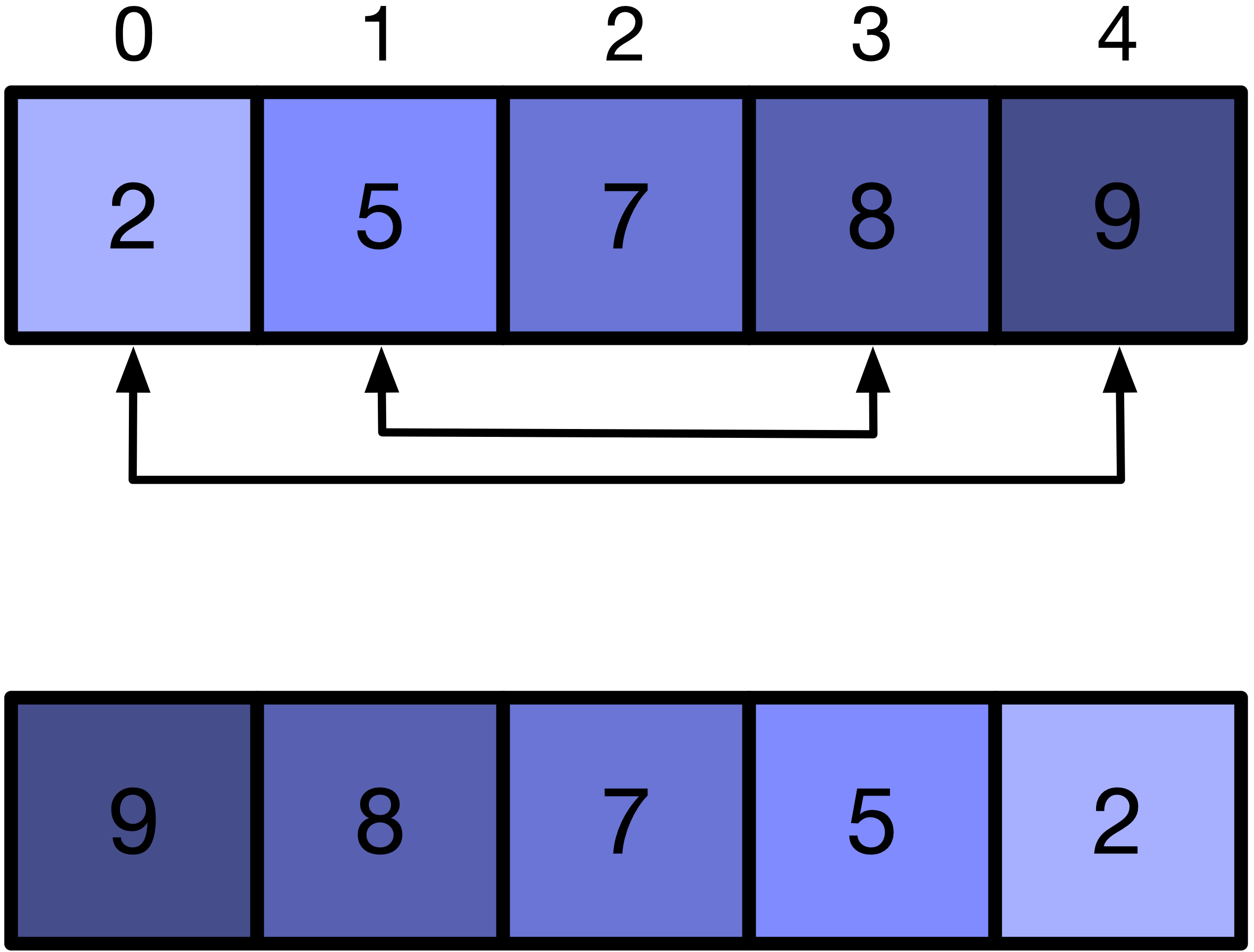# 7.1. Why and how to use arrays?#

Let’s say we have a table containing the grades of all students taking APS 105: Computer Fundamentals course.

Student Name1

100

Whitney Houston

95

Elon Musk

67

Malala Yousafzai

99

Steve Jobs

72

Lionel Messi

101

Mohamed Salah

200

Now, I want to calculate the average of the grades. We can have the grade of each student stored using a int variable. However, if the students were more than 440, as in APS105, I think as an instructor, I will suffer as I type $$440$$+ variable names. Then, I will suffer when summing all these variables to get the average grade.

Dealing with multiple variables is troublesome. Instead, we can have $$\mbox{grade}_1$$, $$\mbox{grade}_2$$, $$\mbox{grade}_3$$ $$\dots$$ stored in one vector named $$\mbox{grade}$$ as we do in math. We can easily then deal with all the grades using the vector of name $$\mbox{grade}$$.

Similarly, in C programming language, we can use an array to represent a vector, elements of a table, contents of boxes in a game, cards in a card deck, weights of a neural network, characters in a word or sentence and many more.

To declare an array that can store $$7$$ grades, we can do the following:Fig. 7.1 Declare an array with $$7$$ int type elements.#

To access and initialize different elements inside the array, we need to index the array as follows:

grades = 100;


In C programming language, to access the first element, we use an index of 0, i.e. first element in the grades array is grades. This makes the last element has an index of one less than the size, i.e. grades is the last element in the grades array. Please note that the size of the array is still $$7$$.

Declare and initialize in the same statement. To declare and initialize an array in the same statement, we can do the following:Fig. 7.2 Declare an array with $$7$$ int type elements, and initialize each element in the array between {} curly brackets.#

Notice in the second way, we did not put the size of the array between [] square brackets, because the compiler will deduce the size of the array from the number of element you are initializing between {} curly brackets. This is only possible when we declare AND initialize in the same statement.

Size of array is fixed!

The size of an array should be a whole number and fixed throughout the entire program. It cannot be shrunk or extended. Hence, the size of the array can be stored in a int, and since the size of the array does not change, we can use const int.

In C programming language, you can also set the size of the array as a macro.

A macro is an alias for a value. It is not a variable. It has no data type. It is not stored in memory. It is only an alias. For example, you can set up a macro as follows:

Code

#define SIZE 7

int main(void) {
int arr[SIZE];
int x = SIZE;
return 0;
}


When the compiler compiles your code, it will replace all SIZE with 7. Hence, the macro SIZE is an alias/nickname for $$7$$. The above code is the same as

Code when compiler replaces all SIZE with 7

int main(void) {
int arr;
int x = 7;
return 0;
}


If you were to set the size of the array as a const int variable, it will look like this:

Code with size of array as const int

int main(void) {
const int Size = 7;
int arr[Size];
int x = Size;
return 0;
}


Given that we now have an array that holds the grades of a class, let’s write a C program that calculates the average of the elements in this array.

Step 1: Toy example. An example array having three elements, int array = {1, 2, 3};. The sum of the elements is $$1 + 2 + 3 = 6$$, and the average is $$\frac{6}{3} = 2$$.

Step 2: Think of a solution! We need to add each element in the array to the summation repeatedly to get the sum of all the elements. Then we divide the summation by the size of the array.

Step 3: Decompose into steps. We need a variable that holds the sum of the array. In each step, we will repeatedly add the elements of the array to sum. Then get the average by dividing sum by the size of the array.

1. Set sum to 0.

2. Set index to 0.

3. Add to sum the element of the array at index.

4. Increment index by $$1$$.

5. Repeat $$3$$$$4$$ until index reaches size of array. If it happens, we shouldn’t add the element at index =  size of the array, since this is beyond the array bounds. The last element to add is at index = size of array - 1.

6. Divide sum by size of array.

Step 4: Write code. Let’s convert the steps into code. Download avg-array.c if you want to run the program yourself.

 1#include <stdio.h>
2#define SIZE 7
3
4int main(void){
5    int grades[SIZE] = {100, 95, 67, 99, 72, 101, 200};
6
7    int sum = 0;
8    double avg = 0;
9
10    for (int index = 0; index < SIZE; index++ ){
11        sum = sum + grades[index];
12    }
13    avg = (double) sum / SIZE;
14    printf("Average is %.2lf", avg);
15    return 0;
16}


Output

Average is 104.86.


Step 5: Debug your code. The above code is working fine for the given example. Some common mistakes that will help you debug your code are:

1. In line $$10$$, instead of going from index = 0 to index = SIZE - 1, you may do the mistake of for(int index = 0; index <= SIZE; index++). Since the condition is index <= SIZE with an equal sign, the index goes from 0 $$\rightarrow$$ SIZE. There is no element at index = SIZE, because the last element is at index = SIZE - 1.

2. In line $$10$$, some people may start from index = 1 to index = SIZE - 1 or to index = SIZE. Starting from index = 1 is missing the first element, which is at index 0. Hence, you MUST start from index = 0. Also, you MUST end at index = SIZE - 1, since there is no element at index SIZE.

3. In line $$13$$, sum is a int and SIZE is a macro with value 7 (no data type). The result of sum / SIZE should be a int with no numbers after the decimal place. Hence, to make the result have numbers after the decimal, we need to type cast sum into double. We learned type cast in Chapter 2.3: Type casting.

Step 6: Test your code. Try out different values in the array, and different array sizes to ensure the code works for different cases.

## 7.1.1. Exercise: Reverse The Elements in an Array#

In this subsection, say we have a few elements in an array that are sorted in ascending order, and we want to reverse these elements as shown in the following figure.

Step 1: Toy example. We can use the above drawing as our example.

Step 2: Think of a solution! We can start by swapping the elements in index $$0$$ and $$5$$, then swap elements at index $$1$$ and $$4$$, then swap elements at index $$2$$ and $$3$$. We shouldn’t swap elements at index $$3$$ and $$2$$, because we just swapped them. This is depicted in the figure above.

Step 3: Decompose into steps.

1. Set variable low to 0 and high to SIZE - 1.

2. Swap element at index low with element at index high.

3. Increment low and decrement high by 1.

4. Repeat $$2$$$$3$$ till all elements are swapped, which happens when low becomes higher than high. But this may not happen if the size of the array is odd, and not even. For example, in the following figure, the size of the array is even. We shouldn’t swap, when low becomes 2 and high becomes 2. Hence, we should stop swapping when low becomes greater than or equal to high.Fig. 7.4 An array with even number of elements before and after reversing.#

Step 4: Write code. Download reverse-array.c if you want to run the program yourself.

 1#include <stdio.h>
2#define SIZE 6
3
4int main(void){
5    int arr[SIZE] = {2, 5, 7, 8, 9, 12};
6    for (int index = 0; index < SIZE; index++){
7        printf("%d, ", arr[index]);
8    }
9    printf("\n");
10    for(int low = 0, high = SIZE - 1; low < high; low++, high--){
11        int temp = arr[low];
12        arr[low] = arr[high];
13        arr[high] = temp;
14    }
15
16    for (int index = 0; index < SIZE; index++){
17        printf("%d, ", arr[index]);
18    }
19    printf("\n");
20    return 0;
21}


Output

2, 5, 7, 8, 9, 12,
12, 9, 8, 7, 5, 2,


In line $$10$$, we initialize low and high in the initialization section of the for loop. Recall in Chapter 4.3 Variations in for loop, we can do so. Similarly, in the increment statement, we incremented low and decremented high.

In lines $$11$$$$13$$, we did what we did in the swap function in Chapter 6.3: How to use pointers to communicate more with functions?.

Step 5: Test your code. Test your code with odd number of elements, one element, and two elements to ensure it would work in different settings.

## 7.1.2. Summary of Important Features of Arrays#

1. First element exists at index at $$0$$.

2. Size of the array is fixed throughout the program.

3. If you are declaring and initializing, you don’t need to put the size between [], because the compiler will deduce the size from the number of elements between {}

What happens if you give less elements than size? For example,

int array  = {1, 2};


This is also equivalent to int array  = {1, 2, 0, 0, 0};. This means that the remaining elements in the array will be set to $$0$$. It happens if we initialize fewer elements than the size of the array.

What happens if you give more elements than size? For example,

int array  = {1, 2, 3, 4, 5, 6};


You will get a warning when you compile your code. The warning will say “warning: excess elements in array initializer”. This is because you are initializing an excess element in the array. If you run the program, you may get an undefined behavior. Possible errors include segmentation fault, bus error or normal operation.

4. If you try accessing an element at an index greater than the SIZE of the array, the behavior is undefined. You may get a segmentation fault, or you may access a memory space having garbage value. Hence, please ensure that the value of the variable you are using as an index is between $$0$$ and the size of the array - 1.

1

All names referred here are fictional character names, and any similarities between these names and reality is probably by coincidence.